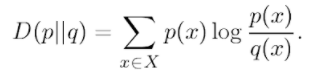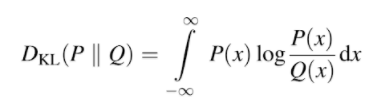# Kullback–Leibler KL Divergence

Kullback–Leibler divergence (also called KL divergence, relative entropy information gain or information divergence) is a way to compare differences between two probability distributions p(x) and q(x). More specifically, the KL divergence of q(x) from p(x) measures how much information is lost when q(x) is used to approximate p(x). It answers the question: If I used the “not-quite” right distribution q(x) to approximate p(x), how many bits of information do I need to more accurately represent p(x)?

## KL Divergence Formula

The formula for the divergence of two discrete probability distributions, defined over a random variable x ∈ X, is as follows:Where:

• X is the set of all possible variables for x.

The log function is sharp close to zero, so this may allow sensitive detection of small probability distribution changes (Sugiyama, 2015).

For continuous probability distributions, the formula (Tyagi, 2018) involves integral calculus:## KL Distance

KL divergence is sometimes called the KL distance (or a “probabilistic distance model”), as it represents a “distance” between two distributions. However, it isn’t a traditional metric (i.e. it isn’t a unit of length). Firstly, it isn’t symmetric in p and q; In other words, the distance from P to Q is different from the distance from Q to P. In addition, it doesn’t satisfy the triangle inequality (Manning & Schütze, 1999).

## References

Ganascia, J. et al. (2008). Discovery Science: 15th International Conference, DS 2012, Lyon, France, October 29-31, 2012, Proceedings. Springer.
Han, J. (2008). Kullback-Leibler Divergence. Retrieved March 16, 2018 from: http://web.engr.illinois.edu/~hanj/cs412/bk3/KL-divergence.pdf
Kullback, S. & Liebler, R. (1951). On Information and Sufficiency. Annals of Mathematical Statistics. 22(1): 79-86.
Manning, C. and Schütze, H. (1999). Foundations of Statistical Natural Language Processing. MIT Press.
Sugiyama, M. (2015). Introduction to Statistical Machine Learning. Morgan Kaufmann.
Tyagi, V. (2018). Content-Based Image Retrieval: Ideas, Influences, and Current Trends. Springer.

CITE THIS AS:
Stephanie Glen. "Kullback–Leibler KL Divergence" From StatisticsHowTo.com: Elementary Statistics for the rest of us! https://www.statisticshowto.com/kl-divergence/